# Fitting functions with PyCaret

This post deals with the approximation of real mathematical functions to one or more real variables using PyCaret without writing code but only acting on the command line of Python scripts that implement the functionality of:

The code described by this post requires Python version 3 and uses the PyCaret library; it also requires the NumPy, Pandas, nad MatPlotLib libraries.
To get the code please see the paragraph Download of the complete code at the end of this post.

For the generation of synthetic training and test datasets, the following common tools (available in the repository) will be used:
• fx_gen.py for the real-valued scalar functions of one real-valued variable $f \colon [a,b] \to {\rm I\!R}$
• fxy_gen.py for the real-valued scalar functions of two real-valued variables $f(x,y) \colon [a,b] \times [c,d] \to {\rm I\!R}$
Also for the visualization of the results, and precisely for the comparison of the test dataset with the prediction, the following common tools (always available in the repository) will be used:

## Configuration of general parameters of PyCaret regressor and training

This chapter presents the program: fit_func.py that implements in sequence five calls to as many functions of the PyCaret library:

• regression.setup which initializes the training environment and creates the transformation pipeline.
• regression.compare_models which trains and evaluates the performance of all available estimators in the model library using cross-validation.
Note: in general compare_models returns a grid of average scores from the various cross-validated estimators; the fit_func.py program will use the best performing estimator, namely the one in the first row of the grid.
• regression.tune_model which tunes the hyperparameters of a given estimator; the fit_func.py program will apply tune_model to the best estimator found by compare_models in the previous step.
• regression.finalize_model that trains a data estimator on the entire data set; the fit_func.py program will apply finalize_model to the estimator returned by tune_model in the previous step.
• regression.save_model saves the transformation pipeline and the trained model object to a file in pickle format for later use by predict_func.py.
The purpose of this program is to execute the above sequence of commands to allow PyCaret to choose the best machine learning algorithm to perform regression to approximate functions without having to write code but acting only on the command line.
In fact through the --setupparams argument the user passes a series of hyperparameters to configure the regression of PyCaret and through the argument --compareparams instead the user passes a series of hyperparameters to the comparison between the algorithms made by PyCaret. Besides the hyperparameters of the underlying PyCaret library, the program supports its own arguments to allow the user to pass the training dataset and the file where to save the trained model.

The program is of type M.I.S.O., that is Multiple Input Simple Output: is designed to approximate a function of the form $f \colon \rm I\!R^n \to \rm I\!R$.
The format of the input dataset is in csv format (with header); one of these columns (indicated by a mandatory argument in the command line) is the target column (the one related to the dependent variable) while all other columns contain the values of the independent variables.

### Usage of the fit_func.py program

To get the program usage you can run this following command:

$python fit_func.py --help and the output got is: usage: fit_func.py [-h] [--version] --trainds TRAIN_DATASET_FILENAME --targetcol TARGET_COLUMN --modelout MODEL_FILE [--metric METRIC] [--setupparams SETUP_PARAMS] [--compareparams COMPARE_PARAMS] fit_func.py fits a multiple-input single-output function dataset using the best regressor chosen by PyCaret optional arguments: -h, --help show this help message and exit --version show program's version number and exit --trainds TRAIN_DATASET_FILENAME Train dataset file (csv format) --targetcol TARGET_COLUMN Target column name --modelout MODEL_FILE Output model file --metric METRIC metric to evaluate the best model --setupparams SETUP_PARAMS Parameters of PyCaret regression.setup function --compareparams COMPARE_PARAMS Parameters of PyCaret regression.compare_models function Where: • -h, --help: shows the usage of the program and ends the execution. • --version: shows the version of the program and ends the execution. • --trainds: path (relative or absolute) of a csv file (with header) that contains the dataset to be used for the training; this file can be generated synthetically e.g. via the program fx_gen.py. or be a dataset actually obtained by measuring a scalar and real phenomenon that depends on a single real-valued variable. • --targetcol: name of the column representing the dependent variable. • --modelout: path (relative or absolute) to a file where to save the trained model in pickle format. • --metric: The metrics to be used in sorting the scoring grid. Allowed values are: MAE, MSE, RMSE, R2, RMSLE, MAPE; the default is R2. • --setupparams: list of parameters to pass to the function regression.setup below; see documentation of regression.setup. • --compareparams: list of parameters to pass to the function regression.compare_models below; see documentation of regression.compare_models ## Prediction and error calculation In this chapter the program predict_func.py is presented and which purpose is to make predictions on a test dataset applying it to a previously selected and trained model by PyCaret via the program fit_func.py always without having to write code but only through the command line. In fact, this program supports arguments through which the user passes the previously selected and trained model, the test dataset and the error measurements to be calculated between the predictions and the true values. The format of the incoming test datasets is identical to that of the training programs mentioned above; obviously here the target column (that of dependent variables) is only used to compare the predicted values with the true values by calculating passed error measurements. ### Usage of the predict_func.py program To get the program usage you can run this following command: $ python predict_func.py --help
and the output got is:

usage: predict_func.py [-h] [--version] --model MODEL_FILE --ds DF_PREDICTION
--targetcol TARGET_COLUMN --predictionout
PREDICTION_DATA_FILE
[--measures MEASURES [MEASURES ...]]

predict_func.py makes prediction of the values of a multiple-input single-
output function with the best pretrained regressor chosen by PyCaret

optional arguments:
-h, --help            show this help message and exit
--version             show program's version number and exit
--model MODEL_FILE    model file
--ds DF_PREDICTION    dataset file (csv format)
--targetcol TARGET_COLUMN
Target column name
--predictionout PREDICTION_DATA_FILE
prediction data file (csv format)
--measures MEASURES [MEASURES ...]
List of built-in sklearn regression measures to
compare prediction with input dataset
Where:
• -h, --help: shows the usage of the program and ends the execution.

• --version: shows the version of the program and ends the execution.

• --model: path (relative or absolute) to the file in pickle format of the model selected and trained by PyCaret and generated by the program mentioned above.

• --ds: path (relative or absolute) of the csv file (with header) that contains the input dataset on which to calculate the prediction.

• --targetcol: name of the column representing the dependent variable.

• --predictionout: path (relative or absolute) of the csv file to generate that will contain the prediction, that is the approximation of the function applied to the input dataset.

• --measures: list of measurements to be calculated by comparing the true values of the input dataset and the predicted output values; the list of supported metrics is defined in SciKit Learn Regression Metrics.

## Examples of approximation of real-valued scalar function of a real-valued variable

### Example #1

Suppose you want to approximate the function $$f(x)=\frac {1}{2} x^3 - 2 x^2 - 3 x - 1$$ in the range $[-10,10]$. The translation of this function in lambda body Python syntax is:

0.5*x**3 - 2*x**2 - 3*x - 1
To generate the training dataset, by the fx_gen.py program, run the following command:

$python fx_gen.py \ --dsout mytrain.csv \ --funcx "0.5*x**3 - 2*x**2 - 3*x - 1" \ --xbegin -10.0 \ --xend 10.0 \ --xstep 0.01 instead to generate the test dataset, run the following command: $ python fx_gen.py \
--dsout mytest.csv \
--funcx "0.5*x**3 - 2*x**2 - 3*x - 1" \
--xbegin -10.0 \
--xend 10.0 \
--xstep 0.0475
Note that the discretization step of the test dataset is larger than that of training and it is a normal fact because the training, to be accurate, it must be run on more data. Also note that it is appropriate for the discretization step of the test dataset is not a multiple of the training one in order to ensure that the test dataset contains most of the data that is not present in training dataset, and this makes prediction more interesting.
To this we intend to make a regression by fit_func.py program passing to the underlying regressor the following arguments: 'train_size': 0.8, 'session_id': 987654321, 'log_experiment': True, 'experiment_name': 'example1_pycaret'; then run the following command:

$python fit_func.py \ --trainds mytrain.csv \ --targetcol y \ --metric MAE \ --modelout mymodel.pickle \ --setupparams "'train_size': 0.8, 'session_id': 987654321, 'log_experiment': True, 'experiment_name': 'example1_pycaret'" and at the end of the execution the program shows the best regression algorithm selected by PyCaret, which is in this experiment ExtraTreesRegressor and saves the file mymodel.pickle which contains the model of the selected, configured and trained regressor. Now we intend to perform the prediction and calculation of the error using the measurements mean_absolute_error and mean_squared_error by the predict_func.py program; then execute the following command: $ python predict_func.py \
--model mymodel.pickle \
--ds mytest.csv \
--targetcol y \
--predictionout mypred.csv \
--measures mean_absolute_error mean_squared_error
and at the end of the execution the saved mypred.csv file contains the prediction performed by applying the model on the test data; the output of the program displays the error measures passed through the argument --measures and are very low: the first around $0.0161$ and the second around $0.002$.
Finally you want to make the comparative display of the test dataset with the prediction by the fx_scatter.py program; therefore run the following command:

$python fx_scatter.py \ --ds mytest.csv \ --prediction mypred.csv \ --xlabel "x" \ --ylabel "y=1/2 x^3 - 2x^2 - 3x - 1" that shows the dispersion graphs of the test dataset and the superimposed prediction: in blue the one of the test dataset, in red the prediction. The comparison of the two graphs clearly shows that the approximation has reached very high levels.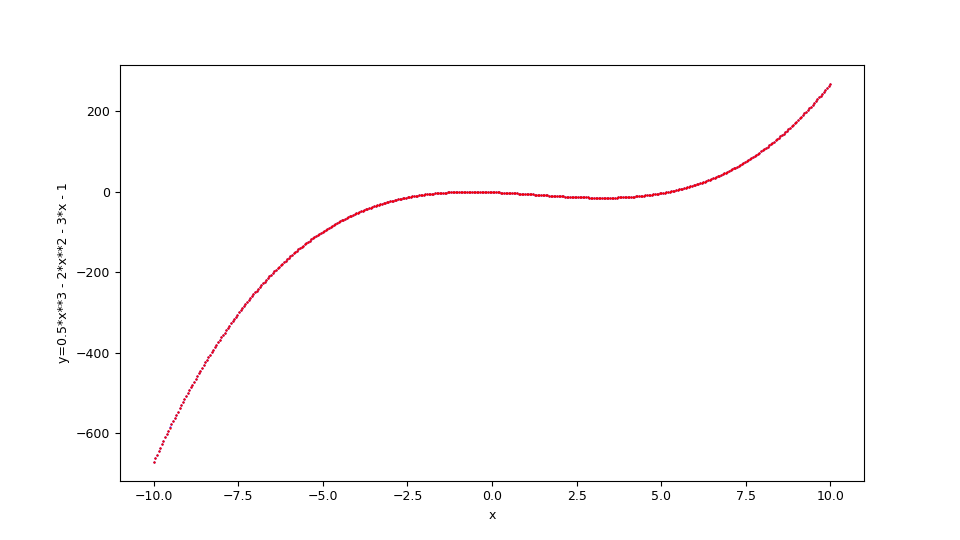Figure with dispersion graphs showing the fitting in red overlay of the function$f(x)=\frac {1}{2} x^3 - 2 x^2 - 3 x - 1$and the original function below in blue. The shell script of this example (that show the use of these cascading programs) at the following url: one-variable-function/pycaret/examples/example1.sh. ### Example #2 Suppose you want to approximate the function $$f(x)=\sin x$$ in the range$[-6,6]$. Keeping in mind that np is the alias of NumPy library, the translation of this function in lambda body Python syntax is: np.sin(x) Executing the same steps as in the previous example, at the end the scatter plots of the test dataset and the prediction are shown superimposed: in blue that of the test dataset, in red the prediction. The comparison of the two graphs clearly shows that the approximation has reached very high levels.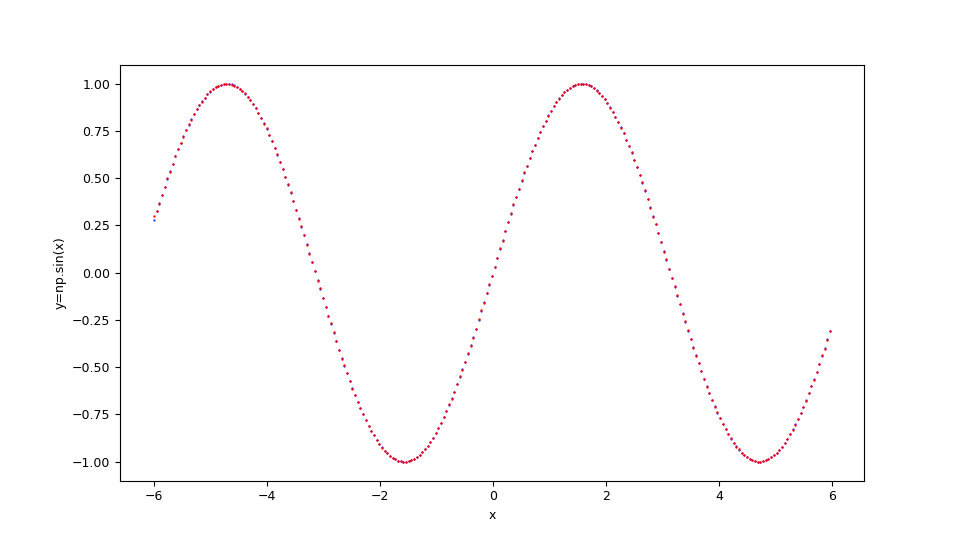Figure with dispersion graphs showing the fitting in red overlay of the function$f(x)=\sin x $and the original function below in blue. The shell script of this example (that show the use of these cascading programs) at the following url: one-variable-function/pycaret/examples/example2.sh. ### Example #3 Suppose you want to approximate the function $$f(x)=e^{\sin x}$$ in the range$[-8,8]$. Keeping in mind that np is the alias of NumPy library, the translation of this function in lambda body Python syntax is: np.exp(np.sin(x)) Executing the same steps as in the previous example, at the end the scatter plots of the test dataset and the prediction are shown superimposed: in blue that of the test dataset, in red the prediction. The comparison of the two graphs clearly shows that the approximation has reached very high levels.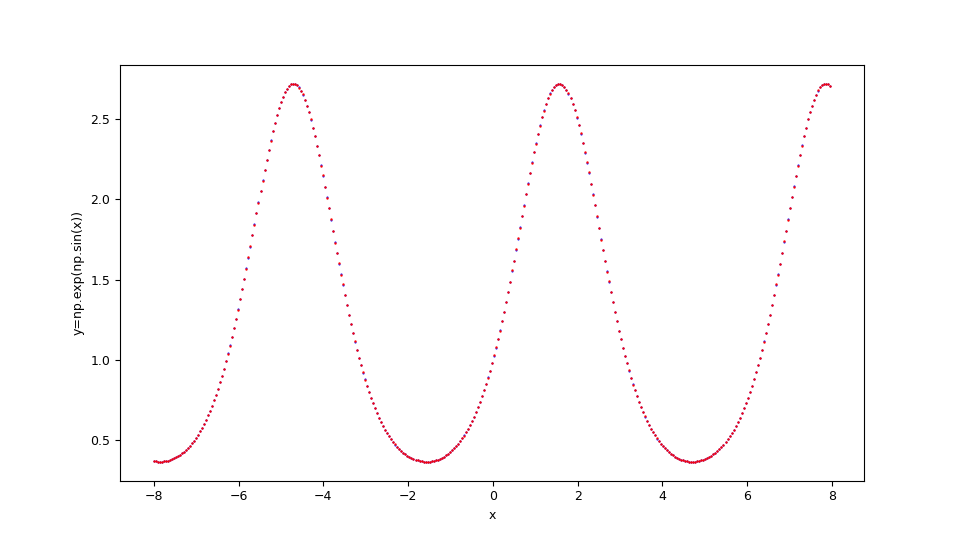Figure with dispersion graphs showing the fitting in red overlay of the function$f(x)=e^{\sin x}$and the original function below in blue. The shell script of this example (that show the use of these cascading programs) at the following url: one-variable-function/pycaret/examples/example3.sh. ## Examples of approximation of real-valued scalar function of two real-valued variables ### Example #1 Suppose you want to approximate the function $$f(x,y)=x^2 + y^2$$ in the set$[-5,5] \times [-5,5]$. The translation of this function in lambda body Python syntax is: x**2 + y**2 To generate the training dataset, by the fxy_gen.py program, run the following command: $ python fxy_gen.py \
--dsout mytrain.csv \
--funcxy "x**2 + y**2" \
--xbegin -3.0 --xend 3.0 \
--ybegin -3.0 --yend 3.0 \
--xstep 0.05 --ystep 0.1
instead to generate the test dataset, run the following command:

$python fyx_gen.py \ --dsout mytest.csv \ --funcxy "x**2 + y**2" \ --xbegin -3.0 --xend 3.0 \ --ybegin -3.0 --yend 3.0 \ --xstep 0.0875 --ystep 0.5 Note that the discretization step of the test dataset is larger than that of training and it is a normal fact because the training, to be accurate, it must be run on more data. Also note that it is appropriate for the discretization step of the test dataset is not a multiple of the training one in order to ensure that the test dataset contains most of the data that is not present in training dataset, and this makes prediction more interesting. To this we intend to make a regression by fit_func.py program passing to the underlying regressor the following arguments: 'train_size': 0.8, 'session_id': 987654321, 'log_experiment': True, 'experiment_name': 'example1_pycaret'; then run the following command: $ python fit_func.py \
--trainds mytrain.csv \
--targetcol z \
--metric MAE \
--modelout mymodel.pickle \
--setupparams "'train_size': 0.8, 'session_id': 987654321,
'log_experiment': True, 'experiment_name': 'example1_pycaret'"
and at the end of the execution the program shows the best regression algorithm selected by PyCaret, which is in this experiment ExtraTreesRegressor and saves the file mymodel.pickle which contains the model of the selected, configured and trained regressor.

Now we intend to perform the prediction and calculation of the error using the measurements mean_absolute_error and mean_squared_error by the predict_func.py program; then execute the following command:

$python predict_func.py \ --model mymodel.pickle \ --ds mytest.csv \ --targetcol z \ --predictionout mypred.csv \ --measures mean_absolute_error mean_squared_error and at the end of the execution the saved mypred.csv file contains the prediction performed by applying the model on the test data; the output of the program displays the error measures passed through the argument --measures and are very low: the first around$0.00389864$and the second around$0.00004255$. Finally you want to make the comparative display of the test dataset with the prediction by the fxy_scatter.py program; therefore run the following command: $ python fxy_scatter.py \
--ds mytest.csv \
--prediction mypred.csv \
--xlabel "x" \
--ylabel "y" \
--zlabel "z=x**2 + y**2"
that shows alongside the dispersion graphs of the test dataset and the prediction: in blue the one of the test dataset, in red the prediction. The comparison of the two graphs clearly shows that the approximation has reached very high levels.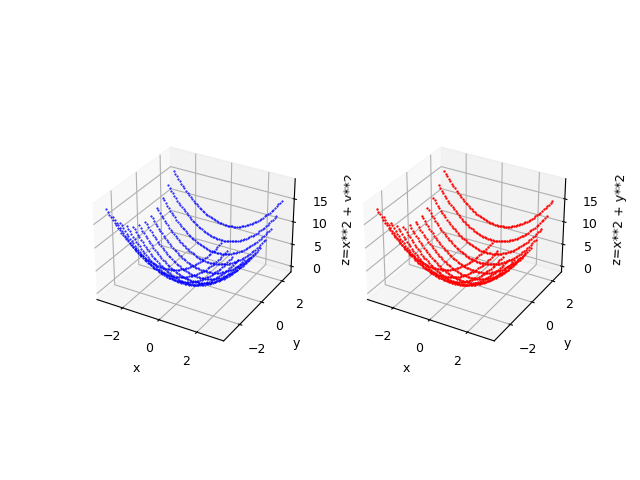Figure with dispersion graphs showing the fitting in red overlay of the function
$f(x,y)=x^2 + y^2$ and alongside the original function in blue.

The shell script of this example (that show the use of these cascading programs) at the following url:
two-variables-function/pycaret/examples/example1.sh.

### Example #2

Suppose you want to approximate the function $$f(x,y)=\sin \sqrt{x^2 + y^2}$$ in the range $[-5,5] \times [-5,5]$. Keeping in mind that np is the alias of NumPy library, the translation of this function in lambda body Python syntax is:

np.sin(np.sqrt(x**2 + y**2))
Executing the same steps as in the previous example, at the end the scatter plots of the test dataset and the prediction are shown alongside: in blue that of the test dataset, in red the prediction. The comparison of the two graphs clearly shows that the approximation has reached very high levels.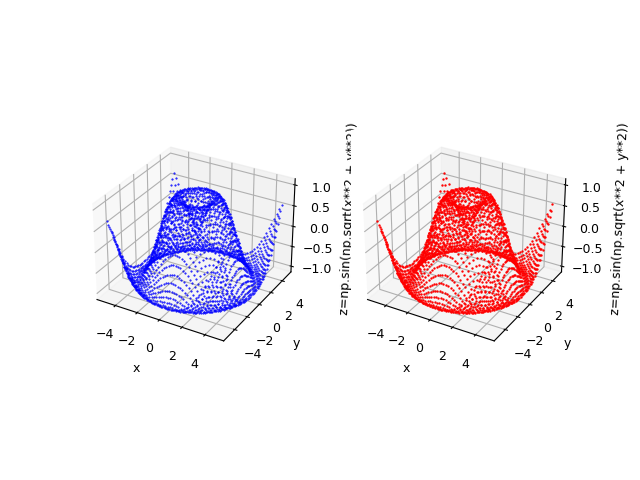Figure with dispersion graphs showing alongside the fitting in red overlay of the function
$f(x,y)=\sin \sqrt{x^2 + y^2}$ and the original function below in blue.

The shell script of this example (that show the use of these cascading programs) at the following url:
two-variables-function/pycaret/examples/example2.sh.

## Citations

@ManualPyCaret,
author  = {Moez Ali},
title   = {PyCaret: An open source, low-code machine learning library in Python},
year    = {2020},
month   = {April},
note    = {PyCaret version 1.0.0},
url     = {https://www.pycaret.org}